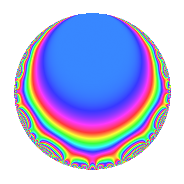# Properties

 Label 71.1.b.aLevel 71 Weight 1 Character orbit 71.b Self dual Yes Analytic conductor 0.035 Analytic rank 0 Dimension 3 Projective image $$D_{7}$$ CM disc. -71 Inner twists 2

# Related objects

## Newspace parameters

 Level: $$N$$ = $$71$$ Weight: $$k$$ = $$1$$ Character orbit: $$[\chi]$$ = 71.b (of order $$2$$ and degree $$1$$)

## Newform invariants

 Self dual: Yes Analytic conductor: $$0.0354336158969$$ Analytic rank: $$0$$ Dimension: $$3$$ Coefficient field: $$\Q(\zeta_{14})^+$$ Coefficient ring: $$\Z[a_1, a_2]$$ Coefficient ring index: $$1$$ Projective image $$D_{7}$$ Projective field Galois closure of 7.1.357911.1 Artin image size $$14$$ Artin image $D_7$ Artin field Galois closure of 7.1.357911.1

## $q$-expansion

Coefficients of the $$q$$-expansion are expressed in terms of a root $$\beta$$ of the polynomial $$x^{3} - x^{2} - 2 x + 1$$. We also show the integral $$q$$-expansion of the trace form.

 $$f(q)$$ $$=$$ $$q -\beta q^{2} + ( 1 + \beta - \beta^{2} ) q^{3} + ( -1 + \beta^{2} ) q^{4} + ( -2 + \beta^{2} ) q^{5} + ( -1 + \beta ) q^{6} + ( 1 - \beta^{2} ) q^{8} + ( 1 - \beta ) q^{9} +O(q^{10})$$ $$q -\beta q^{2} + ( 1 + \beta - \beta^{2} ) q^{3} + ( -1 + \beta^{2} ) q^{4} + ( -2 + \beta^{2} ) q^{5} + ( -1 + \beta ) q^{6} + ( 1 - \beta^{2} ) q^{8} + ( 1 - \beta ) q^{9} + ( 1 - \beta^{2} ) q^{10} - q^{12} + ( -2 - \beta + \beta^{2} ) q^{15} + \beta q^{16} + ( -\beta + \beta^{2} ) q^{18} -\beta q^{19} + ( 1 + \beta ) q^{20} + q^{24} + ( 2 + \beta - \beta^{2} ) q^{25} + ( -1 + \beta ) q^{27} + ( 1 + \beta - \beta^{2} ) q^{29} + q^{30} - q^{32} -\beta q^{36} -\beta q^{37} + \beta^{2} q^{38} + ( -1 - \beta ) q^{40} + ( -2 + \beta^{2} ) q^{43} - q^{45} + ( 1 - \beta ) q^{48} + q^{49} - q^{50} + ( \beta - \beta^{2} ) q^{54} + ( -1 + \beta ) q^{57} + ( -1 + \beta ) q^{58} + ( 2 - \beta^{2} ) q^{60} + q^{71} + \beta q^{72} + ( -2 + \beta^{2} ) q^{73} + \beta^{2} q^{74} + ( 3 - \beta^{2} ) q^{75} + ( 1 - \beta - \beta^{2} ) q^{76} + ( -2 + \beta^{2} ) q^{79} + ( -1 + \beta^{2} ) q^{80} + ( -1 - \beta + \beta^{2} ) q^{81} -\beta q^{83} + ( 1 - \beta^{2} ) q^{86} + ( 2 - \beta ) q^{87} + ( 1 + \beta - \beta^{2} ) q^{89} + \beta q^{90} + ( 1 - \beta^{2} ) q^{95} + ( -1 - \beta + \beta^{2} ) q^{96} -\beta q^{98} +O(q^{100})$$ $$\operatorname{Tr}(f)(q)$$ $$=$$ $$3q - q^{2} - q^{3} + 2q^{4} - q^{5} - 2q^{6} - 2q^{8} + 2q^{9} + O(q^{10})$$ $$3q - q^{2} - q^{3} + 2q^{4} - q^{5} - 2q^{6} - 2q^{8} + 2q^{9} - 2q^{10} - 3q^{12} - 2q^{15} + q^{16} + 4q^{18} - q^{19} + 4q^{20} + 3q^{24} + 2q^{25} - 2q^{27} - q^{29} + 3q^{30} - 3q^{32} - q^{36} - q^{37} + 5q^{38} - 4q^{40} - q^{43} - 3q^{45} + 2q^{48} + 3q^{49} - 3q^{50} - 4q^{54} - 2q^{57} - 2q^{58} + q^{60} + 3q^{71} + q^{72} - q^{73} + 5q^{74} + 4q^{75} - 3q^{76} - q^{79} + 2q^{80} + q^{81} - q^{83} - 2q^{86} + 5q^{87} - q^{89} + q^{90} - 2q^{95} + q^{96} - q^{98} + O(q^{100})$$

## Character Values

We give the values of $$\chi$$ on generators for $$\left(\mathbb{Z}/71\mathbb{Z}\right)^\times$$.

 $$n$$ $$7$$ $$\chi(n)$$ $$-1$$

## Embeddings

For each embedding $$\iota_m$$ of the coefficient field, the values $$\iota_m(a_n)$$ are shown below.

For more information on an embedded modular form you can click on its label.

Label $$\iota_m(\nu)$$ $$a_{2}$$ $$a_{3}$$ $$a_{4}$$ $$a_{5}$$ $$a_{6}$$ $$a_{7}$$ $$a_{8}$$ $$a_{9}$$ $$a_{10}$$
70.1
 1.80194 0.445042 −1.24698
−1.80194 −0.445042 2.24698 1.24698 0.801938 0 −2.24698 −0.801938 −2.24698
70.2 −0.445042 1.24698 −0.801938 −1.80194 −0.554958 0 0.801938 0.554958 0.801938
70.3 1.24698 −1.80194 0.554958 −0.445042 −2.24698 0 −0.554958 2.24698 −0.554958
 $$n$$: e.g. 2-40 or 990-1000 Significant digits: Format: Complex embeddings Normalized embeddings Satake parameters Satake angles

## Inner twists

Char. orbit Parity Mult. Self Twist Proved
1.a Even 1 trivial yes
71.b Odd 1 CM by $$\Q(\sqrt{-71})$$ yes

## Hecke kernels

There are no other newforms in $$S_{1}^{\mathrm{new}}(71, [\chi])$$.